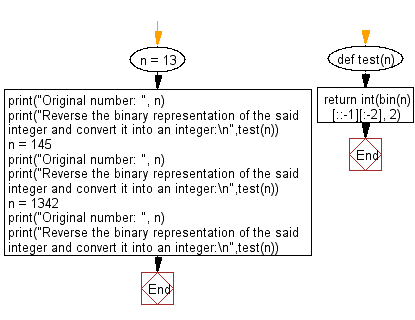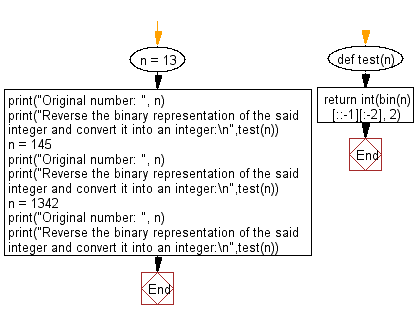﻿ Python: Reverse the binary representation of an integer and convert it into an integer - w3resource# Python: Reverse the binary representation of an integer and convert it into an integer

## Python Basic - 1: Exercise-138 with Solution

Write a Python program to reverse the binary representation of an given integer and convert the reversed binary number into an integer.

Sample Solution-1:

Python Code:

``````def test(n):
return int(bin(n)[::-1][:-2], 2)

n = 13
print("Original number: ", n);
print("Reverse the binary representation of the said integer and convert it into an integer:\n",test(n));
n = 145
print("Original number: ", n);
print("Reverse the binary representation of the said integer and convert it into an integer:\n",test(n));
n = 1342
print("Original number: ", n);
print("Reverse the binary representation of the said integer and convert it into an integer:\n",test(n));
``````

Sample Output:

```Original number:  13
Reverse the binary representation of the said integer and convert it into an integer:
11
Original number:  145
Reverse the binary representation of the said integer and convert it into an integer:
137
Original number:  1342
Reverse the binary representation of the said integer and convert it into an integer:
997
```

Flowchart:## Visualize Python code execution:

The following tool visualize what the computer is doing step-by-step as it executes the said program:

Sample Solution-2:

Python Code:

``````def test(n):
return int(bin(n)[::-1][:-2], 2)
n = 13
print("Original number: ", n);
print("Reverse the binary representation of the said integer and convert it into an integer:\n",test(n));
n = 145
print("Original number: ", n);
print("Reverse the binary representation of the said integer and convert it into an integer:\n",test(n));
n = 1342
print("Original number: ", n);
print("Reverse the binary representation of the said integer and convert it into an integer:\n",test(n));
``````

Sample Output:

```Original number:  13
Reverse the binary representation of the said integer and convert it into an integer:
11
Original number:  145
Reverse the binary representation of the said integer and convert it into an integer:
137
Original number:  1342
Reverse the binary representation of the said integer and convert it into an integer:
997
```

Flowchart:## Visualize Python code execution:

The following tool visualize what the computer is doing step-by-step as it executes the said program:

Python Code Editor:

Have another way to solve this solution? Contribute your code (and comments) through Disqus.

What is the difficulty level of this exercise?

Test your Programming skills with w3resource's quiz.

﻿

## Python: Tips of the Day

What is the difference between Python's list methods append and extend?

append: Appends object at the end.

```x = [1, 2, 3]
x.append([4, 5])
print (x)
```

Output:

```[1, 2, 3, [4, 5]]
```

extend: Extends list by appending elements from the iterable.

```x = [1, 2, 3]
x.extend([4, 5])
print (x)
```

Output:

```[1, 2, 3, 4, 5]
```

Ref: https://bit.ly/2AZ6ZFq